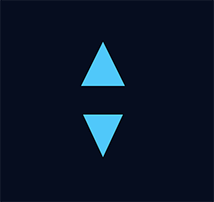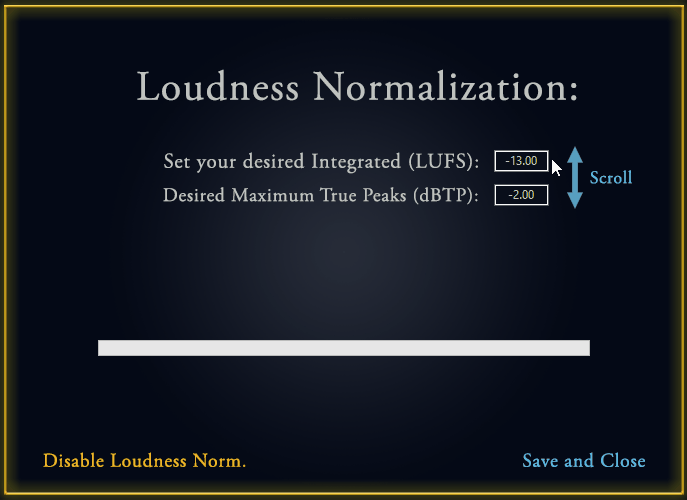# Draw triangle pointing down

Hi guys,
I’m totally lost in the Graphics.FillRectangle, I’m trying to draw two rectangles, one pointing up one pointing down.
It took me about a minute to create the first one (pointing up) but whatever I do I can’t figure out who for crying out loud do I draw the opposite.
This is what I have for the first one (with a little bit of transparency)

```g.DrawingColor = Color.RGB(80, 200, 250, 75) Var points(6) As Double points(1) = 0 points(2) = 45 points(3) = 19 points(4) = 19 points(5) = 40 points(6) = 55 g.FillPolygon(points)```
This is what I wish to end up with:Is it applicable?

That code doesnt produce either of those triangles.

try this:

[quote]var points(6) As Double
points(1) = 37
points(2) = 0
points(3) = 25
points(4) = 25
points(5) = 50
points(6) = 25
g.FillPolygon(points)

points(1) = 37
points(2) = 60
points(3) = 25
points(4) = 35
points(5) = 50
points(6) = 35
g.FillPolygon(points)[/quote]

Amazing, thank you so much!

Just for closure, here is the result so far, there’s still much to do but it starting to look sweet